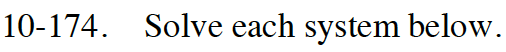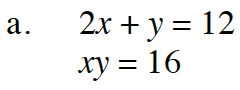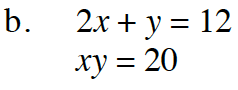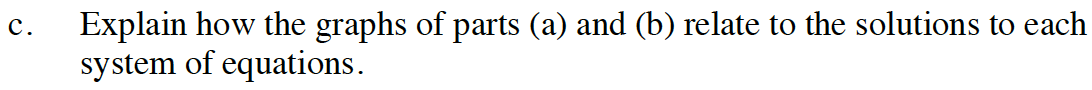### Home > INT3 > Chapter 10 > Lesson 10.3.2 > Problem10-174

10-174.
1. Solve each system below. 10-174 HW eTool (Desmos). Homework Help ✎

1. 2x + y = 12
xy = 16

2. 2x + y = 12
xy = 20

3. Explain how the graphs of parts (a) and (b) relate to the solutions to each system of equations.Note that other sequences of steps are possible.

When x = 4, y = 4, so (4, 4) is a solution.
When x = 2, y = 8, so (2, 8) is a solution.

Now try part (b) on your own.

y = 12 − 2x

x(12 − 2x) = 16

12x − 2x2 − 16 = 0

x2 − 6x + 8 = 0

(x − 4)(x − 2) = 0

x = 4 or x = 2Use the eTool below to help you with this problem.
Click the link at right for the full version of the eTool: INT3 10-174 HW eTool.